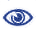# Prague Stringology Conference 2013

Maximal Palindromic Factorization

 Abstract: A palindrome is a symmetric string, phrase, number, or other sequence of units sequence that reads the same forward and backward. We present an algorithm for maximal palindromic factorization of a finite string by adapting an Gusfield algorithm (D. GUSFIELD: Algorithms on strings, trees, and sequences: computer science and computational bioogy, Cambridge University Press, New York, NY, USA, 1997.) for detecting all occurrences of maximal palindromes in a string in linear time to the length of the given string then using the breadth first search (BFS) to find the maximal palindromic factorization set. A factorization of s with respect to refers to a decomposition of s such that s = si1si2···si ℓ where si j ∈ and ℓ is minimum. In this context the set is referred to as the factorization set. In this paper, we tackle the following problem. Given a string s, find the maximal palindromic factorization of s, that is a factorization of s where the factorization set is the set of all center-distinct maximal palindromes of a string s (s).

 Download paper:PostScript PDF BibTeX reference
 Download presentation: